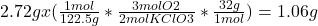## For the unbalanced reaction below, calculate how many grams of oxygen form when 2.72 g of potassium chlorate completely reacts.

Question

For the unbalanced reaction below, calculate how many grams of oxygen form when 2.72 g of potassium chlorate completely reacts.

KClO3(s) –> KCl(s) + O2(g)

in progress 0
6 months 2021-07-14T07:40:07+00:00 1 Answers 10 views 0

## Answers ( )

1.06g O2

Explanation:

Let’s first balance the equation.

2KClO3 > 2KCl + 3O2

We’re going to go from:

1. Grams of KClO3: 2.72g
2. to moles, using molar mass of KClO3: 122.5g/mol
3. We’ll convert to O2 using a mole ratio, where we have 2 mols of KClO3 to 3 mols of O2.
4. To grams of O2 using molar mass: 32g/mol